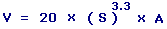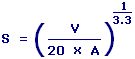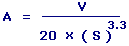Vented Ported Subwoofer Box Calculators Enter value, select unit and click on calculate. Result will be displayed.

Calculate Enclosure Volume or for Box
Calculate Speaker Total at fs
Calculate Air Compliance

 Web www.calculatoredge.com

Calculate Enclosure Volume or for BoxV = Enclosure Volume or Box S = Speaker Total at fs A = Air Compliance
 Enter your values: Speaker Total at fs: Air Compliance: Meter^3 Centimeter^3 Foot^3 Inch^3 Kilometer^3 Yard^3 Liter Milliliter Deciliter Cord Firewood Cord Foot Wood Gallon UK Gallon US Liquid Ounce UK Fluid Ounce US Fluid Quart US Result: Enclosure Volume or Box: Meter3

Calculate Speaker Total at fsS = Speaker Total at fs V = Enclosure Volume or Box A = Air Compliance
 Enter your values: Enclosure Volume or Box: Meter^3 Centimeter^3 Foot^3 Inch^3 Kilometer^3 Yard^3 Liter Milliliter Deciliter Cord Firewood Cord Foot Wood Gallon UK Gallon US Liquid Ounce UK Fluid Ounce US Fluid Quart US Air Compliance: Meter^3 Centimeter^3 Foot^3 Inch^3 Kilometer^3 Yard^3 Liter Milliliter Deciliter Cord Firewood Cord Foot Wood Gallon UK Gallon US Liquid Ounce UK Fluid Ounce US Fluid Quart US Result: Speaker Total at fs:

Calculate Air ComplianceA = Air Compliance V = Enclosure Volume or Box S = Speaker Total at fs
 Enter your values: Enclosure Volume or Box: Meter^3 Centimeter^3 Foot^3 Inch^3 Kilometer^3 Yard^3 Liter Milliliter Deciliter Cord Firewood Cord Foot Wood Gallon UK Gallon US Liquid Ounce UK Fluid Ounce US Fluid Quart US Speaker Total at fs: Result: Air Compliance: Meter3LINKSDISCLAIMERCONTACT US